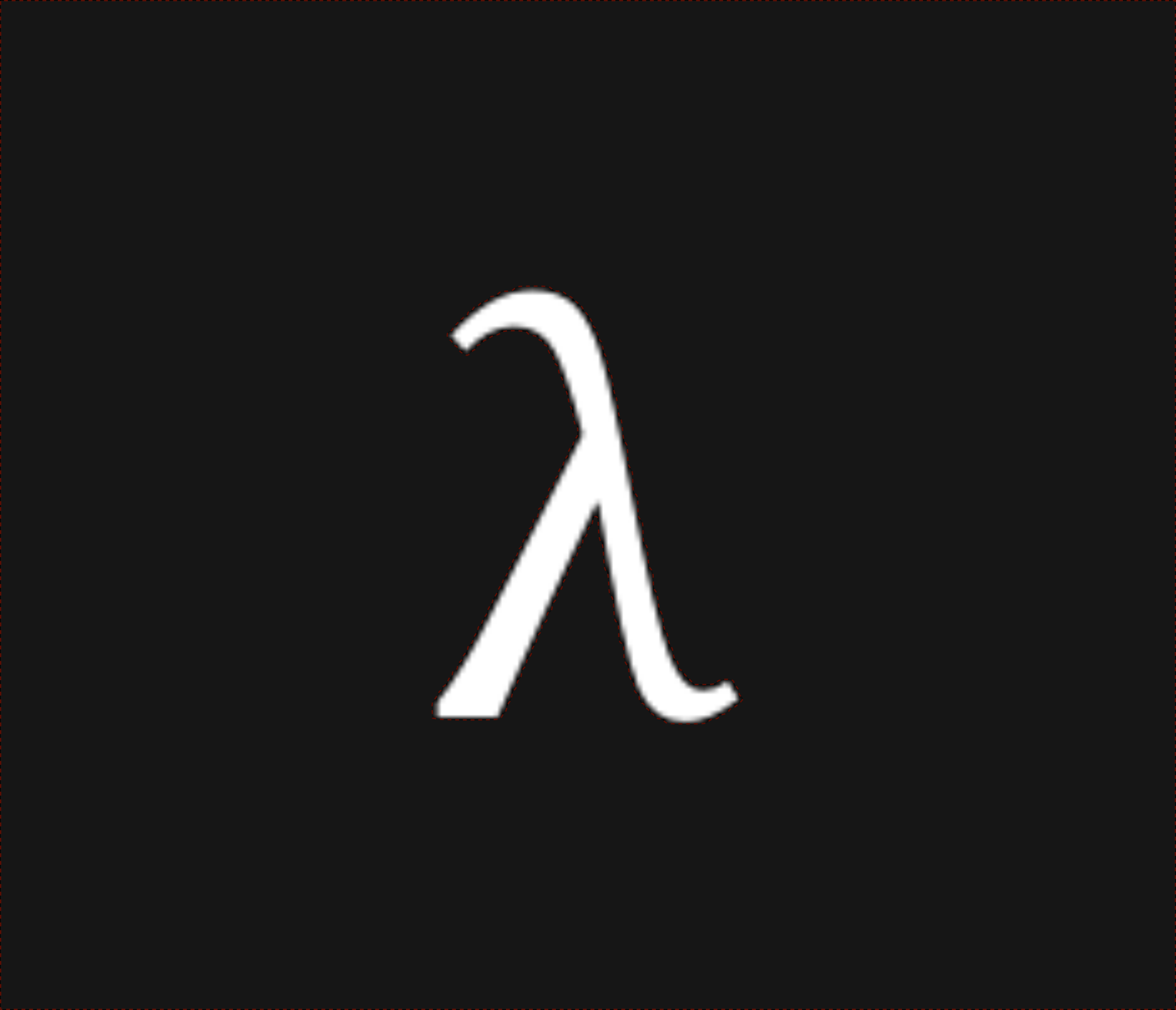nixpulvis

## Wish 202 words written by Nathan Lilienthal

Time passes from event to event before resolution.

(... e1 e2)

$\epsilon_1 \cdots \epsilon_2$

A promise always resolves itself.

(... (. e) e)

$\cdot\epsilon \ \cdots \ \epsilon$

A try can fail.

(... (\ e) (e | f))

$\dot\epsilon \ \cdots \ \epsilon \ | \ \lambda$

A wish might resolve.

(... (e .) (| e))

$\epsilon\cdot \ \cdots \ | \ \epsilon$

A lie always fails if resolved.

(... (e \) (| f))

$\underset{\cdot}{\epsilon} \ \cdots \ | \ \lambda$

I try to wish to $$\epsilon$$.

$\dot\epsilon \ \cdots \ \cdot \ | \ \lambda$

I wish to try to $$\epsilon$$.

$\dot\epsilon\cdot \ \cdots$

I try then lie about $$\epsilon$$.

$\dot\epsilon \ \cdots \ \underset{\cdot}{\epsilon}$

I try $$\epsilon_1$$ then lie about $$\epsilon_2$$.

$\dot\epsilon_1 \ \cdots \ \underset{\cdot}{\epsilon_2}$

I promise to lie to try to wish for $$\epsilon$$.

$\cdot\underset{\cdot}{\dot\epsilon}\cdot$
• $$\cdot$$ kinda fits with context in “I try to wish”.
• Duplicate actions are tricky to write (e.g. “I try to try”).
• Precedence is a mess.
• Must time pass between verbs?